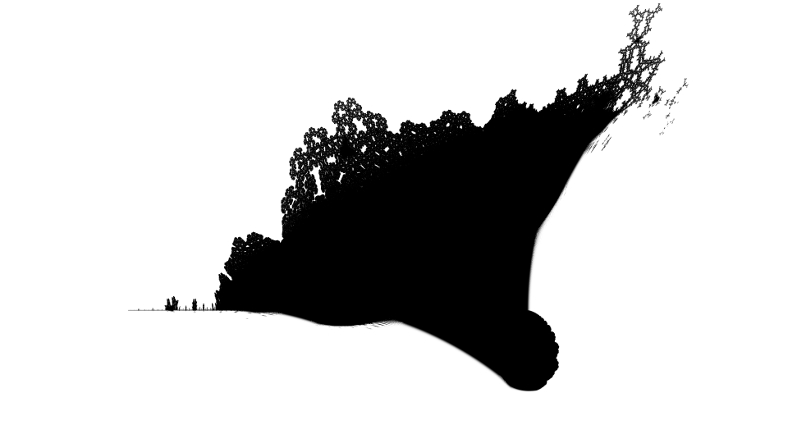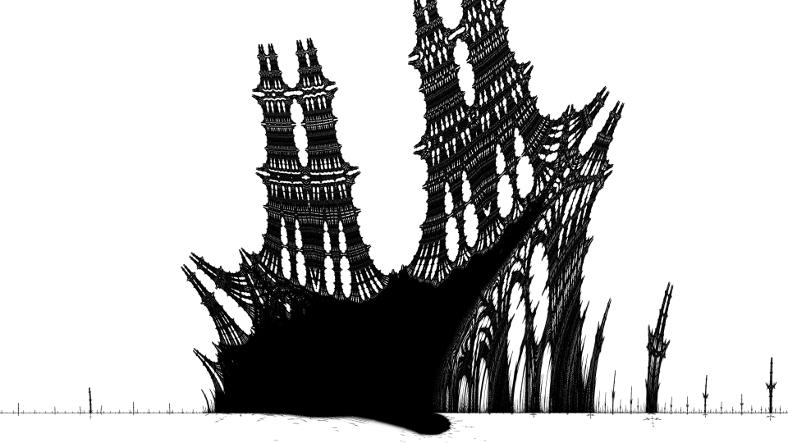# mathr / blog / #

## The Burning ShipThe Burning Ship fractal is defined by iterations of:

\begin{aligned} X &\leftarrow X^2 - Y^2 + A \\ Y &\leftarrow 2|XY| + B \end{aligned}

The Burning Ship set is those points $$A + i B \in \mathbb{C}$$ whose iteration starting from $$X + i Y = 0$$ remains bounded. In practice one iterates a maximum number of times, or until the point diverges (exercise suggested on Reddit: prove a lower bound on an escape radius that is sufficient for the Burning Ship, the Mandelbrot set has the bound $$R = 2$$). Note that traditionally the Burning Ship is rendered with the imaginary $$B$$ axis increasing downwards, which makes the "ship" the right way up.Traditional (continuous) iteration count (escape time) rendering tends to lead to a grainy appearance for this fractal, so I prefer distance estimation. To compute a distance estimate one can use partial derivatives (aka Jacobian matrix):

\begin{aligned} \frac{\partial X}{\partial A} &\leftarrow 2 \left(X \frac{\partial X}{\partial A} - Y \frac{\partial Y}{\partial A}\right) + 1 \\ \frac{\partial X}{\partial B} &\leftarrow 2 \left(X \frac{\partial X}{\partial B} - Y \frac{\partial Y}{\partial B}\right) \\ \frac{\partial Y}{\partial A} &\leftarrow 2 \operatorname{sgn}(X) \operatorname{sgn}(Y) \left( X \frac{\partial Y}{\partial A} + \frac{\partial X}{\partial A} Y \right) \\ \frac{\partial Y}{\partial B} &\leftarrow 2 \operatorname{sgn}(X) \operatorname{sgn}(Y) \left( X \frac{\partial Y}{\partial B} + \frac{\partial X}{\partial B} Y \right) + 1 \end{aligned}

Then the distance estimate for an escaped point is (thanks to gerrit on fractalforums.org):

$d = \frac{||\begin{pmatrix}X & Y\end{pmatrix})||^2 \log ||\begin{pmatrix}X & Y\end{pmatrix}||}{\left|\left|\begin{pmatrix}X & Y\end{pmatrix} \cdot \begin{pmatrix} \frac{\partial X}{\partial A} & \frac{\partial X}{\partial B} \\ \frac{\partial Y}{\partial A} & \frac{\partial Y}{\partial B} \end{pmatrix} \right|\right|}$

Then scale $$d$$ by the pixel spacing, colouring points with small distance dark, and large distance light. I colour interior points dark too.

Perturbation techniques can be used for efficient deep zooms. Compute a high precision orbit of $$A,B,X,Y$$, and have low precision deltas $$a,b,x,y$$ for each pixel. It works out as:

\begin{aligned} x &\leftarrow (2 X + x) x - (2 Y + y) y + a \\ y &\leftarrow 2 \operatorname{diffabs}(XY, Xy + xY + xy) + b \end{aligned}

where $$\operatorname{diffabs}(c, d) = |c + d| - |c|$$ but expanded into case analysis to avoid catastrophic cancellation with limited precision floating point (this is I believe due to laser blaster on fractalforums.com):

$\operatorname{diffabs}(c, d) = \begin{cases} d & c \ge 0, c + d \ge 0 \\ -2c - d & c \ge 0, c + d < 0 \\ 2c + d & c < 0, c + d > 0 \\ -d & c < 0, c + d \le 0 \end{cases}$

Due to the non-analytic functions, series approximation cannot be used. As with perturbation rendering of the Mandelbrot set, glitches can occur. It seems that Pauldelbrot's glitch criterion (originally posted on fractalforums.com) is also applicable, with a glitch when:

$|(X + x) + (Y + y) i|^2 < 10^{-3} |X + i Y|^2$

Glitched pixels can be recalculated with a new reference. It may be beneficial to pick as new references those pixels with the smallest LHS of the glitch criterion. The derivatives for distance estimation don't need to be perturbed as they are not "small", one can use $$X + x$$ etc in the derivative recurrences.

When navigating the Burning Ship, it is noticeable that "mini-ships" occur, being distorted self-similar copies of the whole set. When passing by, embedded Julia sets appear, similarly to the Mandelbrot set, with period doubling when approaching mini-ships. To zoom directly to mini-ships, one can use Newton's method in 2 real variables. First one needs the period, which can be found by iterating the corners of a polygon until it surrounds the origin, that iteration number is the period (this method is due to Robert Munafo's mu-ency, originally for the Mandelbrot set, but seems to work for the Burning Ship too: perhaps the non-conformal folding is sufficiently rare to be unproblematic in practice). Newton's method iterations are like this:

$\begin{pmatrix} A \\ B \end{pmatrix} \leftarrow \begin{pmatrix} A \\ B \end{pmatrix} - \begin{pmatrix} \frac{\partial X}{\partial A} & \frac{\partial X}{\partial B} \\ \frac{\partial Y}{\partial A} & \frac{\partial Y}{\partial B} \end{pmatrix}^{-1} \begin{pmatrix} X \\ Y \end{pmatrix}$

The final part is the mini-ship size estimate, to know how deep to zoom. The Mandelbrot size estimate seems to work with minor modifications to use Jacobian matrices instead of complex numbers.

These concrete equations are specific to the quadratic Burning Ship, but the methods in principle apply to many escape time fractals.